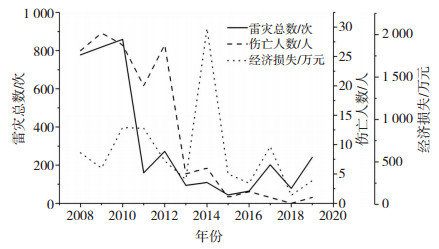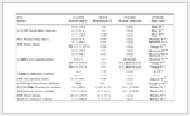﻿ 基于信息扩散理论的江苏省雷电灾害风险评估
 期刊检索： 题目 作者 关键词
 暴雨灾害2021, Vol. 40Issue (5): 564-568.  DOI: 10.3969/j.issn.1004-9045.2021.05.014### DOI

10.3969/j.issn.1004-9045.2021.05.014

### 文章历史

1. 南京信息工程大学气象灾害预报预警与评估协同创新中心/中国气象局气溶胶与云降水重点开放实验室, 南京 210044;
2. 中国气象科学院灾害天气国家重点实验室, 北京 100081;
3. 天津市气象灾害防御技术中心, 天津 300074;
4. 江苏省气象局, 南京 210009

Lightning disaster risk assessment in Jiangsu province based on information diffusion theory
LI Xia1,2, SONG Nannan3, LIU Yinping1 , JIAO Xue4 , YANG Tingya4 , MIU Pei1
1. Key Laboratory for Aerosol-Cloud-Precipitation of China Meteorological Administration, and Collaborative Innovation Center on Forecast and Evaluation of Meteorological Disasters(CIC-FEMD), Nanjing University of Information Science&Technology, Nanjing 210044;
2. State Key Laboratory of Severe Weather, Chinese Academy of Meteorological Sciences, Beijing 100081;
3. Tianjin Meteorological Disaster Defense Technology Centre, Tianjin 300074;
4. Jiangsu Provincial Meteorological Bureau, Nanjing 210009
Abstract: Lightning disaster has been one of the serious disasters affecting the development of economy and society. Based on information diffusion theory, the model of lightning disaster risk assessment is established. And the lightning disasters from 2008 to 2019 in Jiangsu province are analysed by this model. The results show that lightning disasters have been mainly concentrated in 320 times per year, about once every 1.9 years. The probability of more than 880 times per year is low, about once every 38.2 years in Jiangsu province. The risk of life injury and death has been mainly concentrated in 12 people per year, about once every 1.8 years. The number of casualties for more than 30 people per year is about once every 14.1 years. Then the cumulative probability of economic loss with the risk value of 6.50 million yuan per year is 58.62%, but the probability of 22.50 million yuan per year is very low, only 1.84%. Through quantitative calculation and analysis, a deeper understanding of lightning disasters is achieved and the scientific basis for the government departments to make disaster prevention and mitigation decisions is provided.
Key words: information diffusion theory    lightning disaster    risk assessment    probability of occurrence

1 研究方法与资料来源

2 基于信息扩散理论的雷电灾害风险评估模型

 $X=\left\{x_{1}, x_{2}, \ldots, x_{n}\right\}$ (1)

 $P=\left\{p_{1}, p_{2}, \ldots, p_{n}\right\}$ (2)

 $Y=\left\{y_{1}, y_{2}, \ldots, y_{m}\right\}$ (3)

yj (j = 1，2，…，m) 均称为实际发生的某一雷电灾害样本。

 $U=\left\{u_{1}, u_{2}, \ldots, u_{n}\right\}$ (4)

 $f_{j}\left(u_{i}\right)=\frac{1}{h \sqrt{2 \pi}} \exp \left[-\frac{\left(y_{j}-u_{i}\right)^{2}}{2 h^{2}}\right]$ (5)

 $h=\left\{\begin{array}{ll} 0.8146(b-a), & n=5 ; \\ 0.5690(b-a), & n=6 \\ 0.4560(b-a), & n=7 ; \\ 0.3860(b-a), & n=8 ; \\ 0.3362(b-a), & n=9 \\ 0.2986(b-a), & n=10 ; \\ 2.6851(b-a) /(n-1), & n \geq 11 . \end{array}\right\}$ (6)

 $C_{j}=\sum\limits_{i=1}^{n} f_{j}\left(u_{i}\right)$ (7)

 $\mu_{y_{j}}\left(u_{i}\right)=f_{j}\left(u_{i}\right) / C_{j}$ (8)

 $q\left(u_{i}\right)=\sum\limits_{j=1}^{m} \mu_{y_{j}}\left(u_{i}\right)$ (9)

 $Q=\sum\limits_{i=1}^{n} q\left(u_{i}\right)$ (10)

 $P\left(u_{i}\right)=q\left(u_{i}\right) / Q$ (11)

 $P\left(u \geq u_{i}\right)=\sum\limits_{k=i}^{n} P\left(u_{k}\right)$ (12)

3 江苏雷电灾害风险分析图 1 2008—2019年江苏省雷电灾害年际变化特征 Fig. 1 Annual variation characteristics of lightning disasters in Jiangsu province from 2008 to 2019.

 $U_{1}=\left\{u_{11}, u_{12}, \ldots \ldots u_{122}\right\}=\{40, 80, \ldots \ldots .880\}$ (13)

 $U_{2}=\left\{u_{21}, u_{22}, \ldots \ldots u_{211}\right\}=\{0, 3, \ldots \ldots 30\}$ (14)

 $U_{3}=\left\{u_{31}, u_{32}, \ldots \ldots . u_{312}\right\}=\{50, 250, \ldots \ldots .2250\}$ (15)表 1 雷电灾害次数的风险评估 Table 1 The risk assessment of the total number of lightning disasters.表 2 雷电灾害人身伤亡总数的风险评估 Table 2 The risk assessment of the loss of human life by lightning.表 3 雷电灾害经济损失的风险评估 Table 3 The risk assessment of the loss of economic value by lightning.
4 结论与讨论

 成勤, 史雅静, 刘云鹏, 等. 2016. 自然风景区雷电灾害风险评估方法研究[J]. 暴雨灾害, 38(3): 291-296. DOI:10.3969/j.issn.1004-9045.2016.03.013 程向阳, 谢五三, 王凯, 等. 2012. 雷电灾害风险区划方法研究及其在安徽省的应用[J]. 气象科学, 32(1): 79-83. 崔新强, 付佳, 代娟, 等. 2021. 基于Arcgis和AHP的湖北省内高铁(城铁)线路雷电灾害风险区划[J]. 灾害学, 36(2): 80-85. 杜子璇, 刘静, 刘伟昌. 2012. 基于信息扩散理论的长江中下游地区高温热害风险分析[J]. 气象与环境科学, 35(2): 8-14. DOI:10.3969/j.issn.1673-7148.2012.02.002 金晨路, 肖稳安, 王学良. 2011. 湖北省雷电灾害易损性分析与区划[J]. 暴雨灾害, 30(3): 272-276. DOI:10.3969/j.issn.1004-9045.2011.03.012 郦嘉诚, 钟颖颖, 冯民学. 2012. 江苏省区域闪电分布特征[J]. 大气科学学报, 35(3): 380-384. DOI:10.3969/j.issn.1674-7097.2012.03.014 林昕, 管兆勇. 2008. 中国华东地区夏季高温的时空特征和年际变化[J]. 南京气象学院学报, 31(1): 1-9. DOI:10.3969/j.issn.1674-7097.2008.01.001 刘亚彬, 刘黎明, 许迪, 等. 2010. 基于信息扩散理论的中国粮食主产区水旱灾害风险评估[J]. 农业工程学报, 26(8): 1-7. DOI:10.3969/j.issn.1002-6819.2010.08.001 刘引鸽, 缪启龙, 高庆九. 2005. 基于信息扩散理论的气象灾害风险评估方法[J]. 气象科学, 25(1): 84-89. DOI:10.3969/j.issn.1009-0827.2005.01.012 栾健, 李家启, 肖稳安, 等. 2013. 基于信息扩散理论的雷电天气关注度研究[J]. 西南师范大学学报(自然科学版), 38(9): 143-149. DOI:10.3969/j.issn.1000-5471.2013.09.025 王春扬, 杨超. 2010. 信息扩散技术在重大雷灾预测中的应用[J]. 气象科技, 38(2): 270-273. DOI:10.3969/j.issn.1671-6345.2010.02.025 王学林, 黄琴琴, 柳军. 2019. 基于信息扩散理论的南方双季早稻气象灾害风险评估[J]. 中国农业气象, 11(40): 712-722. 王莺, 李耀辉, 赵福年, 等. 2013. 基于信息扩散理论的甘肃省农业旱灾风险分析[J]. 干旱气象, 31(1): 44-48. 王莺, 张强, 韩兰英. 2016. 基于信息扩散理论的中国南方水旱灾害风险特征[J]. 干旱气象, 34(6): 919-926. 魏道江, 李慧民. 2015. 运用投影寻踪及信息扩散理论评价深基坑施工风险[J]. 中国安全科学学报, 25(2): 147-152. 吴安坤, 李忠良, 李艳, 等. 2015. 基于历史灾情数据的雷电灾害风险分析与评价[J]. 防灾科技学院学报, 17(4): 26-31. DOI:10.3969/j.issn.1673-8047.2015.04.004 余田野, 王学良, 袁海锋, 等. 2019. 基于投影寻踪方法的湖北省雷电灾害风险区划[J]. 暴雨灾害, 38(3): 291-296. DOI:10.3969/j.issn.1004-9045.2019.03.012 张丽娟, 李文亮, 张冬有. 2009. 基于信息扩散理论的气象灾害风险评估方法[J]. 地理科学, 29(2): 250-254. DOI:10.3969/j.issn.1000-0690.2009.02.017 赵伟, 杨续超, 张斌, 等. 2014. 浙江省雷电灾害风险分析及区划[J]. 热带气象学报, 30(5): 996-1000. DOI:10.3969/j.issn.1004-4965.2014.05.021 朱浩, 王凯, 程向阳, 等. 2015. 安徽省雷电灾害特征分析及区域灾情评估[J]. 长江流域资源与环境, 24(1): 162-163. DOI:10.11870/cjlyzyyhj201501022 中国气象局. 2017. QX/T103-2017. 雷电灾害调查技术规范[S] Lai Y, Gerald R. 1990. Information Theory and Climate Prediction[J]. Journal of Climate, 3: 514. Liu B, Siu Y, Gordon M, et al. 2013. Exceedance probability of multiple natural hazards: risk assessment in China's Yangtze river delta[J]. Nat Hazards, 69: 2039-2055. DOI:10.1007/s11069-013-0794-8 Wu M, Chen Y, Xu C, et al. 2015. Assessment of meteorological disasters based on information diffusion theory in Xinjiang, Northwest China[J]. Journal of Geographical Sciences, 25(1): 69-84. DOI:10.1007/s11442-015-1154-2 Xu L, Liu G. 2009. The study of a method of regional environmental risk assessment[J]. Journal of Environmental Management, 90: 3290-3296.